Miscellaneous

Chapter 10 Class 7 Practical Geometry
Serial order wise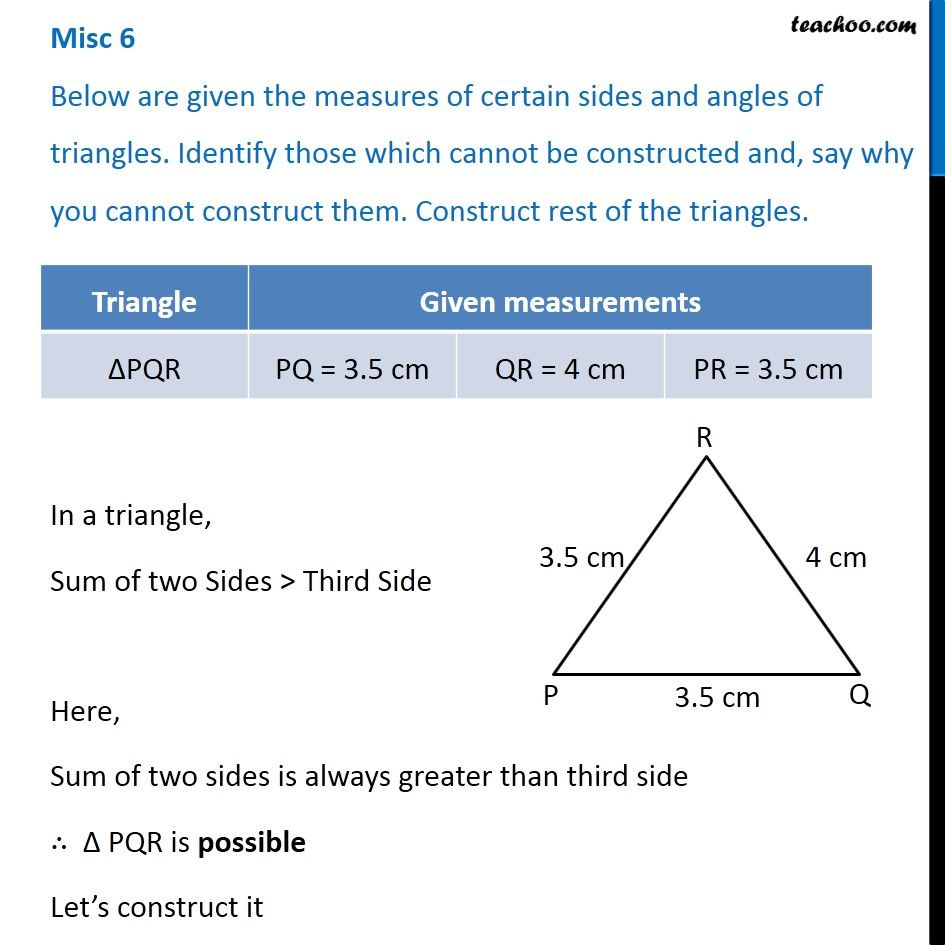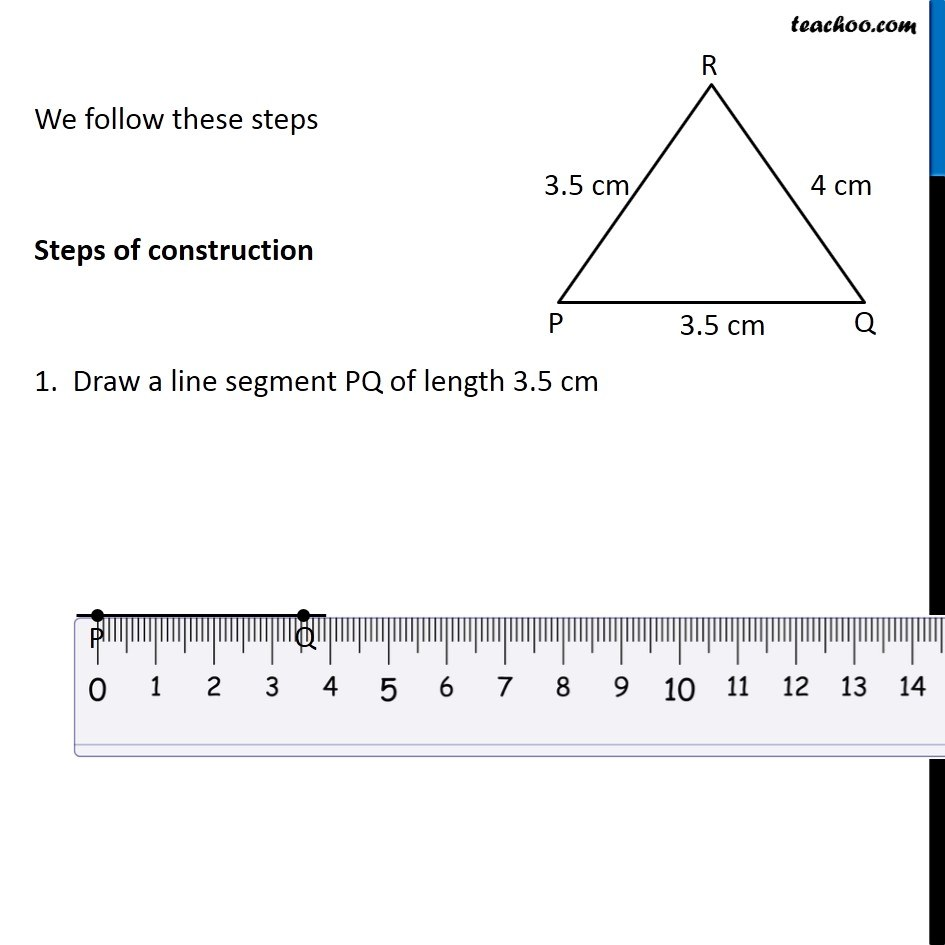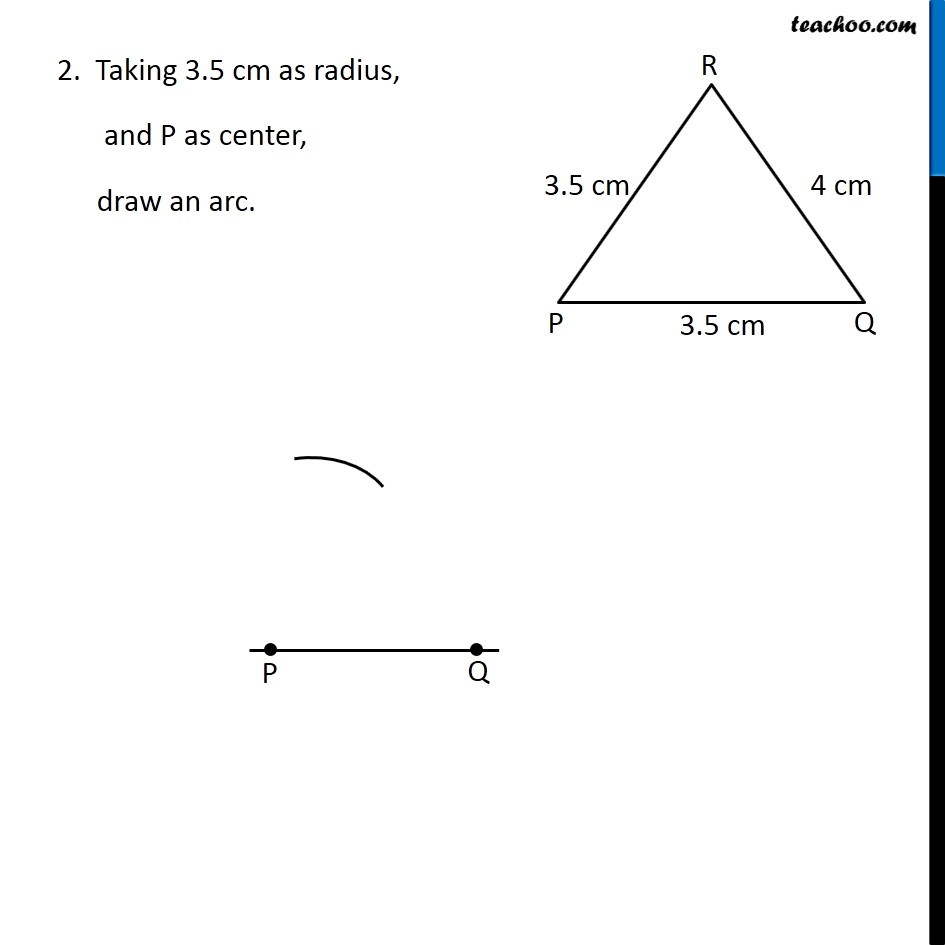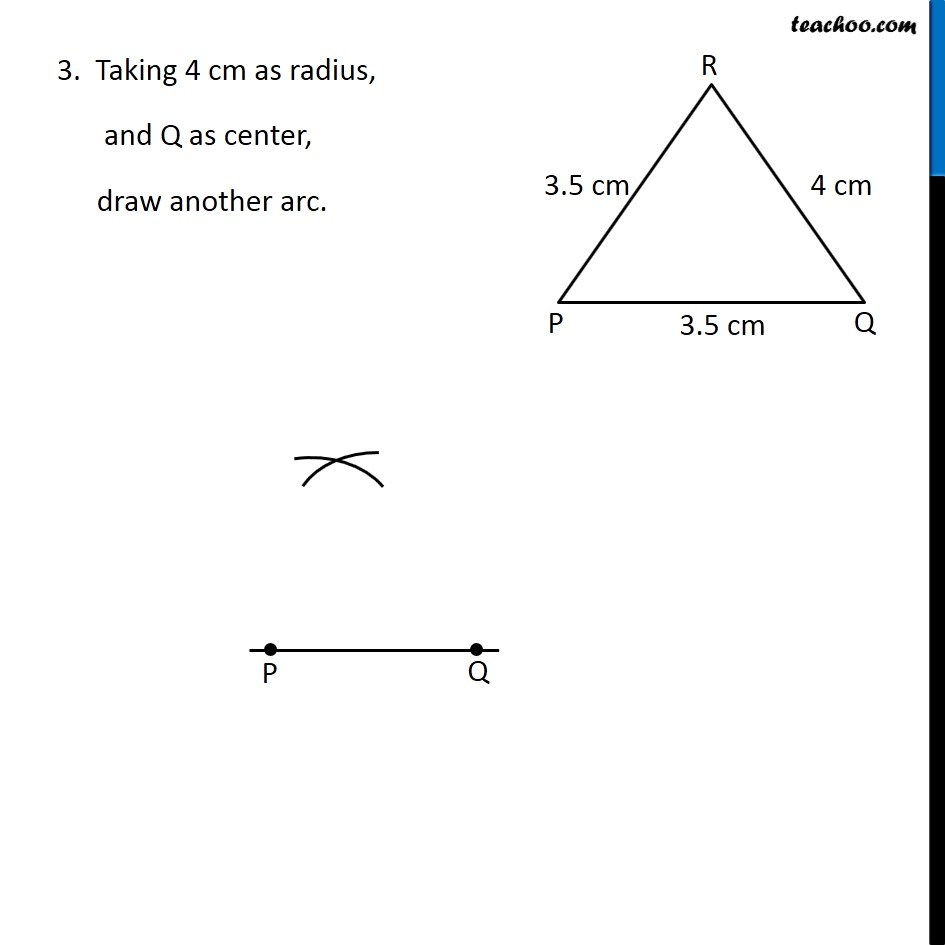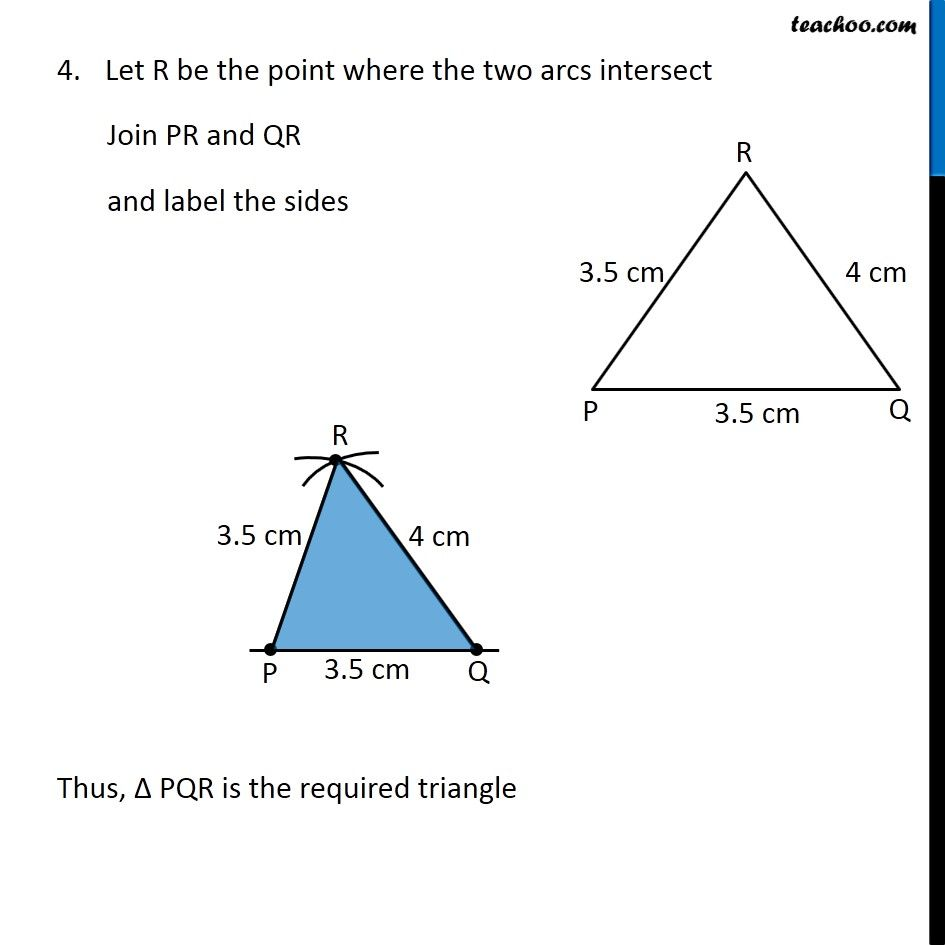### Transcript

Misc 6 Below are given the measures of certain sides and angles of triangles. Identify those which cannot be constructed and, say why you cannot construct them. Construct rest of the triangles. In a triangle, Sum of two Sides > Third Side Here, Sum of two sides is always greater than third side ∴ Δ PQR is possible Let’s construct it We follow these steps Steps of construction 1. Draw a line segment PQ of length 3.5 cm 2. Taking 3.5 cm as radius, and P as center, draw an arc. 3. Taking 4 cm as radius, and Q as center, draw another arc. Let R be the point where the two arcs intersect Join PR and QR and label the sides Thus, Δ PQR is the required triangle